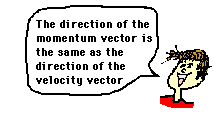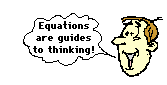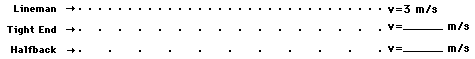Momentum and Its Conservation - Lesson 1 - The Impulse-Momentum Change Theorem

MomentumThe sports announcer says, "Going into the all-star break, the Chicago White Sox have the momentum." The headlines declare "Chicago Bulls Gaining Momentum." The coach pumps up his team at half-time, saying "You have the momentum; the critical need is that you use that momentum and bury them in this third quarter."

Momentum is a commonly used term in sports. A team that has the momentum is on the move and is going to take some effort to stop. A team that has a lot of momentum is really on the move and is going to be hard to stop. Momentum is a physics term; it refers to the quantity of motion that an object has. A sports team that is on the move has the momentum. If an object is in motion (on the move) then it has momentum.Momentum can be defined as "mass in motion." All objects have mass; so if an object is moving, then it has momentum - it has its mass in motion. The amount of momentum that an object has is dependent upon two variables: how much stuff is moving and how fast the stuff is moving. Momentum depends upon the variables mass and velocity. In terms of an equation, the momentum of an object is equal to the mass of the object times the velocity of the object.

Momentum = mass • velocity

In physics, the symbol for the quantity momentum is the lower case p. Thus, the above equation can be rewritten as

p = m • v

where m is the mass and v is the velocity. The equation illustrates that momentum is directly proportional to an object's mass and directly proportional to the object's velocity.

The units for momentum would be mass units times velocity units. The standard metric unit of momentum is the kg•m/s. While the kg•m/s is the standard metric unit of momentum, there are a variety of other units that are acceptable (though not conventional) units of momentum. Examples include kg•mi/hr, kg•km/hr, and g•cm/s. In each of these examples, a mass unit is multiplied by a velocity unit to provide a momentum unit. This is consistent with the equation for momentum.

Momentum as a Vector Quantity

Momentum is a vector quantity. As discussed in an earlier unit, a vector quantity is a quantity that is fully described by both magnitude and direction. To fully describe the momentum of a 5-kg bowling ball moving westward at 2 m/s, youmust include information about both the magnitude and the direction of the bowling ball. It is not enough to say that the ball has 10 kg•m/s of momentum; the momentum of the ball is not fully described until information about its direction is given. The direction of the momentum vector is the same as the direction of the velocity of the ball. In a previous unit, it was said that the direction of the velocity vector is the same as the direction that an object is moving. If the bowling ball is moving westward, then its momentum can be fully described by saying that it is 10 kg•m/s, westward. As a vector quantity, the momentum of an object is fully described by both magnitude and direction.

The Momentum Equation as a Guide to Thinking

From the definition of momentum, it becomes obvious that an object has a large momentum if both its mass and its velocity are large. Both variables are of equal importance in determining the momentum of an object. Consider a Mack truck and a roller skate moving down the street at the same speed. The considerably greater mass of the Mack truck gives it a considerably greater momentum. Yet if the Mack truck were at rest, then the momentum of the least massive roller skate would be the greatest. The momentum of any object that is at rest is 0. Objects at rest do not have momentum - they do not have any "mass in motion." Both variables - mass and velocity - are important in comparing the momentum of two objects.

The momentum equation can help us to think about how a change in one of the two variables might affect the momentum of an object. Consider a 0.5-kg physics cart loaded with one 0.5-kg brick and moving with a speed of 2.0 m/s. The total mass of loaded cart is 1.0 kg and its momentum is 2.0 kg•m/s. If the cart was instead loaded with three 0.5-kg bricks, then the total mass of the loaded cart would be 2.0 kg and its momentum would be 4.0 kg•m/s. A doubling of the mass results in a doubling of the momentum.Similarly, if the 2.0-kg cart had a velocity of 8.0 m/s (instead of 2.0 m/s), then the cart would have a momentum of 16.0 kg•m/s (instead of 4.0 kg•m/s). A quadrupling in velocity results in a quadrupling of the momentum. These two examples illustrate how the equation p = m•v serves as a "guide to thinking" and not merely a "plug-and-chug recipe for algebraic problem-solving."

Express your understanding of the concept and mathematics of momentum by answering the following questions. Click the button to view the answers.

1. Determine the momentum of a ...

a. 60-kg halfback moving eastward at 9 m/s.

b. 1000-kg car moving northward at 20 m/s.

c. 40-kg freshman moving southward at 2 m/s.

2. A car possesses 20 000 units of momentum. What would be the car's new momentum if ...

a. its velocity was doubled.

b. its velocity was tripled.

c. its mass was doubled (by adding more passengers and a greater load)

d. both its velocity was doubled and its mass was doubled.

3. A halfback (m = 60 kg), a tight end (m = 90 kg), and a lineman (m = 120 kg) are running down the football field. Consider their ticker tape patterns below.Compare the velocities of these three players. How many times greater are the velocity of the halfback and the velocity of the tight end than the velocity of the lineman?

Which player has the greatest momentum? Explain.

Next Section: